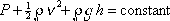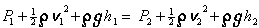This is Bernoulli's equation:

P + one-half rho v-squared + rho g h = constant

Pressure + one-half density times velocity-squared + density times gravitational force constant times the elevation above a reference level, is the same throughout an incompressible fluid in streamline flow through a pipe which is completely full of the fluid.

Tip: To use this equation take advantage of the fact that the quantity on the left side of the equation has the same value at every point in the fluid by setting that quantity at one position in the fluid equal to the same quantity at another point in the fluid:You are provided Bernoulli's equation as written at the top of this page but you need to write it as shown here (just above this line) and use it in this form.